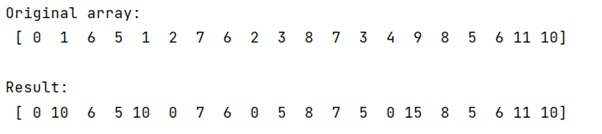# Fast replacement of values in a NumPy array

Learn, how to replace the values of a numpy array using a very fast technique?
Submitted by Pranit Sharma, on February 07, 2023

NumPy is an abbreviated form of Numerical Python. It is used for different types of scientific operations in python. Numpy is a vast library in python which is used for almost every kind of scientific or mathematical operation. It is itself an array which is a collection of various methods and functions for processing the arrays.

## Problem statement

Suppose that we are working on a large numpy array that has millions of values in it and a small dictionary map for replacing some of the elements in this numpy array. Since the numpy array is really a large array hence replacing the values using indexing other functions would be inefficient hence, we need to find a fast method to solve this problem.

## Replacing the values of a numpy array

For this purpose, we will first create a copy of this array and then iterate through the dictionary to replace the values of the array where ever the key matches the values of the array.

Let us understand with a small sized array

## Python code to replace the values of a numpy array using a very fast technique

```# Import numpy
import numpy as np

# Creating a numpy array
arr = np.array([0,1,6,5,1,2,7,6,2,3,8,7,3,4,9,8,5,6,11,10])

# Display original array
print("Original array:\n",arr,"\n")

# Creating a dictionary
d = {4: 0, 3: 5, 1: 10, 9: 15, 2: 0}

# Creating a copy of array
new = np.copy(arr)

# Replacing values
for i,j in d.items():
new[arr==i] = j

# Display result
print("Result:\n",new)
```

### Output

The output of the above program is: